# Trung tâm đào tạo thiết kế vi mạch Semicon

• Đăng ký
 Name: * Tên đăng nhập: * Email: * Mật khẩu: * Verify Password: * Fields marked with an asterisk (*) are required.## Digital Combinational Logic Part-V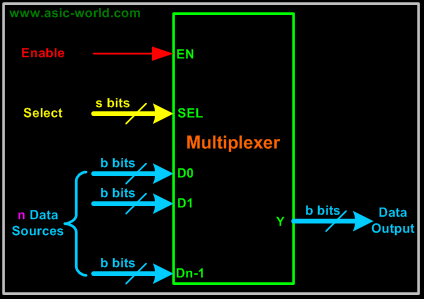Multiplexer
A multiplexer (MUX) is a digital switch which connects data from one of n sources to the output. A number of select inputs determine which data source is connected to the output. The block diagram of MUX with n data sources of b bits wide and s bits wide select line is shown in below figure.MUX acts like a digitally controlled multi-position switch where the binary code applied to the select inputs controls the input source that will be switched on to the output as shown in the figure below. At any given point of time only one input gets selected and is connected to output, based on the select input signal.
Mechanical Equivalent of a Multiplexer

The operation of a multiplexer can be better explained using a mechanical switch as shown in the figure below. This rotary switch can touch any of the inputs, which is connected to the output. As you can see at any given point of time only one input gets transferred to output.

Example - 2x1 MUX
A 2 to 1 line multiplexer is shown in figure below, each 2 input lines A to B is applied to one input of an AND gate. Selection lines S are decoded to select a particular AND gate. The truth table for the 2:1 mux is given in the table below.
Symbol

Truth Table

 S Y 0 A 1 B

Design of a 2:1 Mux

To derive the gate level implementation of 2:1 mux we need to have truth table as shown in figure. And once we have the truth table, we can draw the K-map as shown in figure for all the cases when Y is equal to '1'.

Combining the two 1' as shown in figure, we can drive the output y as shown below

 Y = A.S' + B.S Truth Table B A S Y Â 0 0 0 0 Â 0 0 1 0 Â 0 1 0 1 Â 0 1 1 0 Â 1 0 0 0 Â 1 0 1 1 Â 1 1 0 1 Â 1 1 1 1 Â

Kmap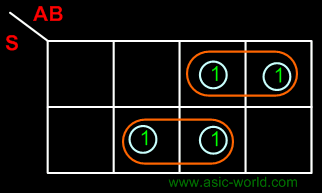Circuit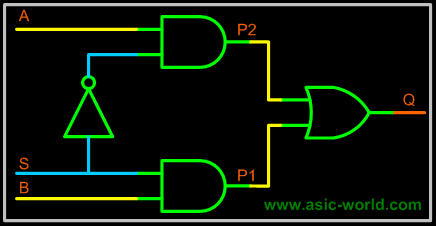Example : 4:1 MUX
A 4 to 1 line multiplexer is shown in figure below, each of 4 input lines I0 to I3 is applied to one input of an AND gate. Selection lines S0 and S1 are decoded to select a particular AND gate. The truth table for the 4:1 mux is given in the table below.
Symbol

ÂTruth Table

.

 S1 S0 Y 0 0 I0 0 1 I1 1 0 I2 1 1 I3

CircuitLarger Multiplexers
Larger multiplexers can be constructed from smaller ones. An 8-to-1 multiplexer can be constructed from smaller multiplexers as shown below.

Example - 8-to-1 multiplexer from Smaller MUX
Truth Table

 S2 S1 S0 F 0 0 0 I0 0 0 1 I1 0 1 0 I2 0 1 1 I3 1 0 0 I4 1 0 1 I5 1 1 0 I6 1 1 1 I7 Circuit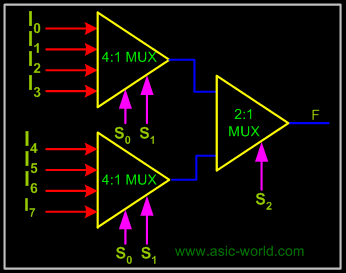. Â . . . .

Example - 16-to-1 multiplexer from 4:1 mux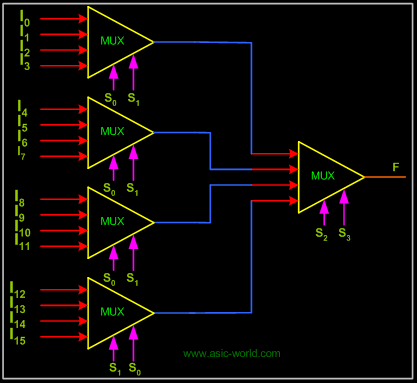Bạn Có Đam Mê Với Vi Mạch hay Nhúng      -     Bạn Muốn Trau Dồi Thêm Kĩ Năng

# Hãy Để Chúng Tôi Hỗ Trợ Cho Bạn. SEMICON

Lần cập nhật cuối ( Thứ ba, 29 Tháng 3 2022 00:12 )